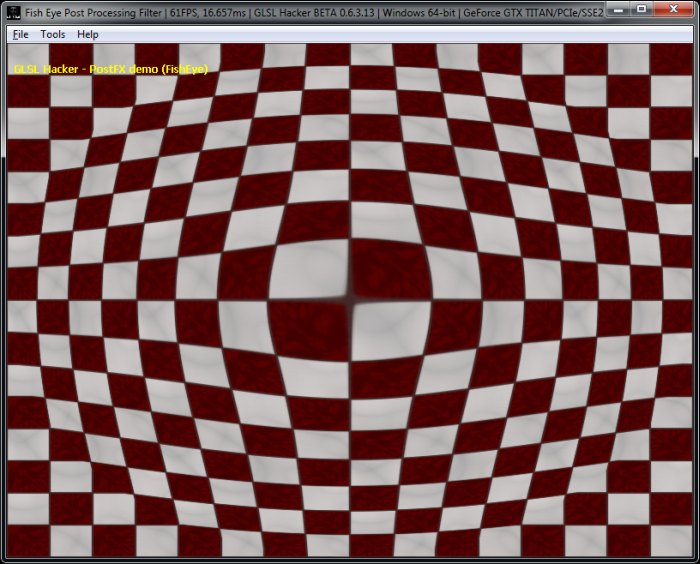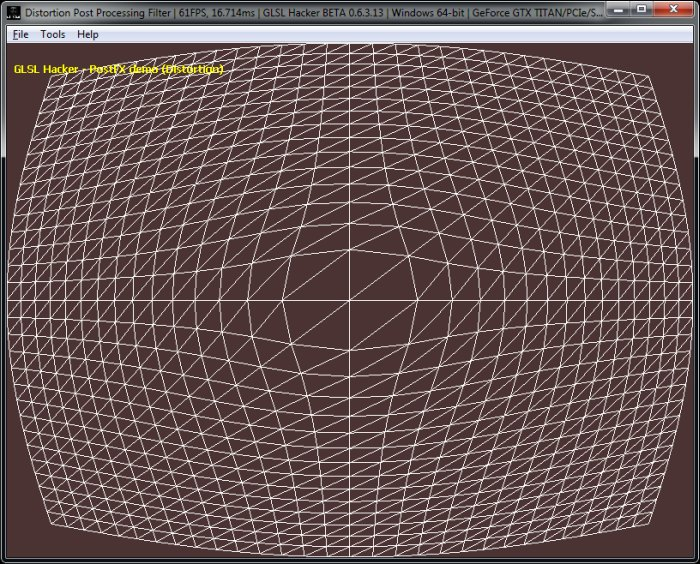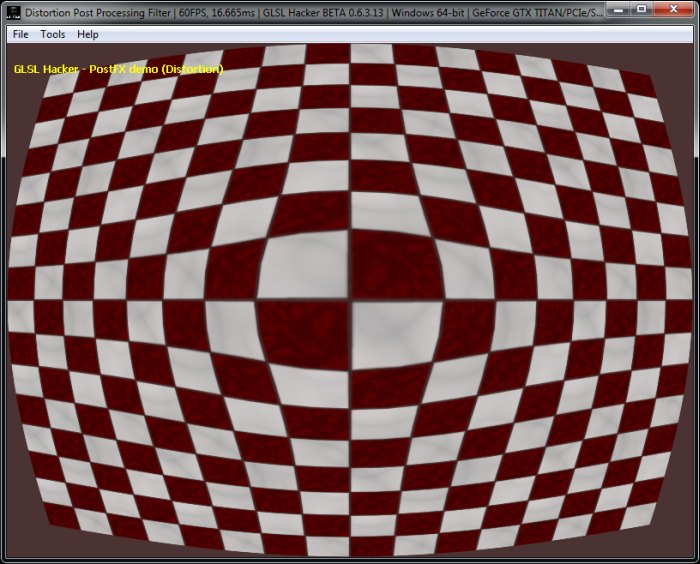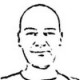# (Shader Library) Fish Eye, Dome and Barrel Distortion GLSL Post Processing Filters

Article index

## 2 – Barrel Distortion Shaders

### 2.1 – Barrel Distortion Pixel ShaderBarrel distortion in the pixel shader

Barrel distortion is a lens effect which causes images to be spherised or inflated (source).

The first barrel distortion demo shows a regular post processing filter that runs the filter in the pixel shader:

```#version 120
varying vec4 Vertex_UV;
uniform mat4 gxl3d_ModelViewProjectionMatrix;
void main()
{
gl_Position = gxl3d_ModelViewProjectionMatrix * gl_Vertex;
Vertex_UV = gl_MultiTexCoord0;
}
```

```#version 120
uniform sampler2D tex0;
varying vec4 Vertex_UV;
const float PI = 3.1415926535;
uniform float BarrelPower;

vec2 Distort(vec2 p)
{
float theta  = atan(p.y, p.x);
return 0.5 * (p + 1.0);
}

void main()
{
vec2 xy = 2.0 * Vertex_UV.xy - 1.0;
vec2 uv;
float d = length(xy);
if (d < 1.0)
{
uv = Distort(xy);
}
else
{
uv = Vertex_UV.xy;
}
vec4 c = texture2D(tex0, uv);
gl_FragColor = c;
}
```

### 2.2 - Barrel Distortion Vertex Shader

In the second barrel distortion demo, the main processing is done in the vertex shader and requires a tessellated fullscreen quad. In the demo, the post processing quad is made up of 30x30 subdivisions:

```#version 120
varying vec4 Vertex_UV;
uniform mat4 gxl3d_ModelViewProjectionMatrix;
uniform float BarrelPower;

vec4 Distort(vec4 p)
{
vec2 v = p.xy / p.w;
// Convert to polar coords:
{
float theta = atan(v.y,v.x);

// Distort:

// Convert back to Cartesian:
p.xy = v.xy * p.w;
}
return p;
}

void main()
{
vec4 P = gxl3d_ModelViewProjectionMatrix * gl_Vertex;
gl_Position = Distort(P);
Vertex_UV = gl_MultiTexCoord0;
}
```

```uniform sampler2D tex0;
varying vec4 Vertex_UV;
uniform int wireframe;

void main()
{
vec4 c = vec4(1.0);
if (wireframe == 0)
{
vec2 uv = Vertex_UV.xy;
c = texture2D(tex0, uv);
}
gl_FragColor = c;
}
```Barrel distortion in the vertex shader - wireframe modeBarrel distortion in the vertex shader - solid + textured mode

Article index

## 2 thoughts on “(Shader Library) Fish Eye, Dome and Barrel Distortion GLSL Post Processing Filters”

1.fenbf